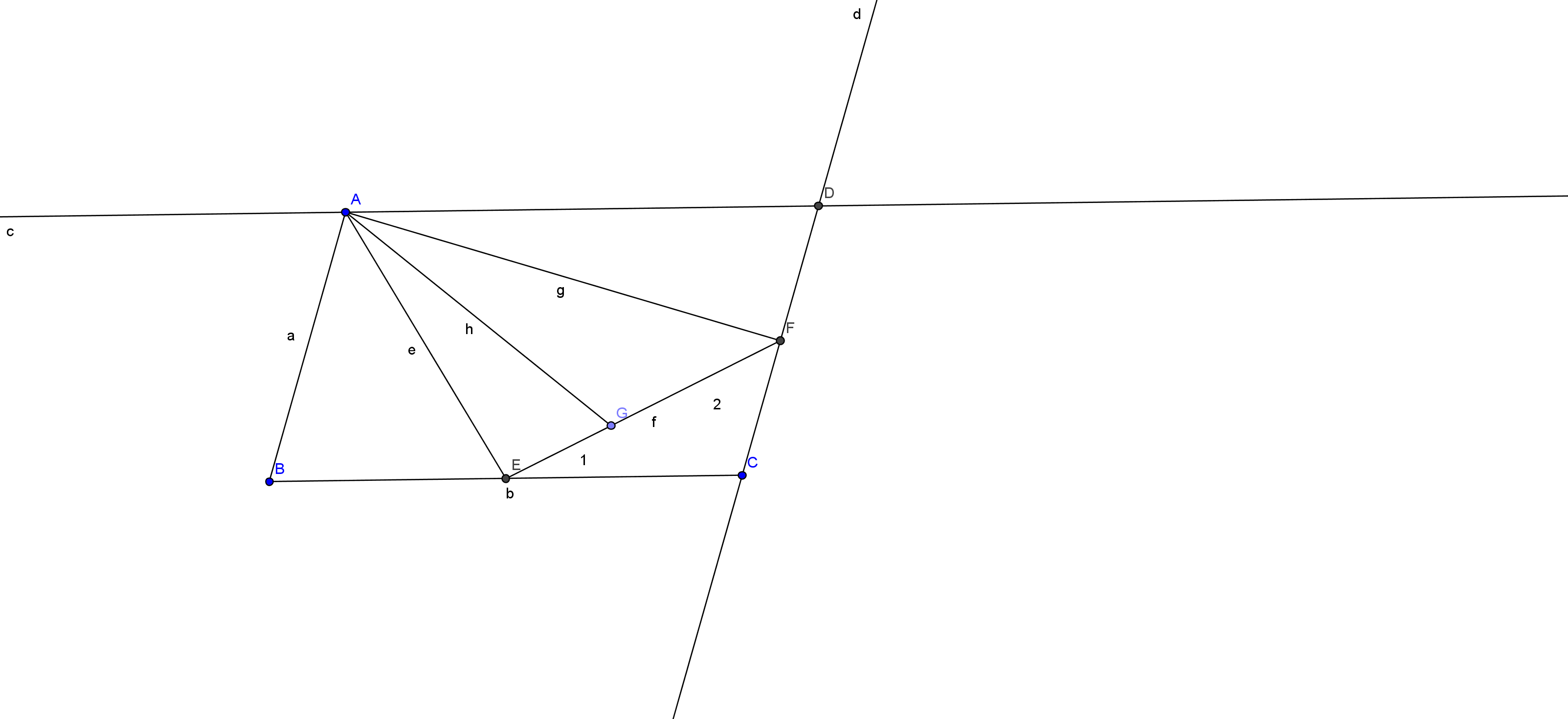# Awesome geometry - 3

Geometry Level 4In parallelogram $ABCD$ shown above, $E$ is the midpoint of $BC$, $F$ is the midpoint of $CD$. $G$ is a point on $EF$ such that ratio $EG : GF$ equal $1 : 2$. If the area of this parallelogram is 776, find Area of triangle $AEG$.

×